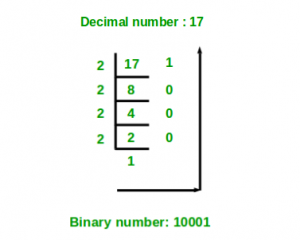# Decimal to binary number using recursion

• Difficulty Level : Easy
• Last Updated : 30 Nov, 2021

Given a decimal number as input, we need to write a program to convert the given decimal number into equivalent binary number.

Examples :

Attention reader! All those who say programming isn't for kids, just haven't met the right mentors yet. Join the  Demo Class for First Step to Coding Coursespecifically designed for students of class 8 to 12.

The students will get to learn more about the world of programming in these free classes which will definitely help them in making a wise career choice in the future.

```Input : 7
Output :111

Input :10
Output :1010```

We have discussed one iterative solution in below post.
Program for Decimal to Binary Conversion
Below is Recursive solution:

```findBinary(decimal)
if (decimal == 0)
binary = 0
else
binary = decimal % 2 + 10 * (findBinary(decimal / 2)```Step by step process for better understanding of how the algorithm works
Let decimal number be 10.
Step 1-> 10 % 2 which is equal-too 0 + 10 * ( 10/2 ) % 2
Step 2-> 5 % 2 which is equal-too 1 + 10 * ( 5 / 2) % 2
Step 3-> 2 % 2 which is equal-too 0 + 10 * ( 2 / 2 ) % 2
Step 4-> 1 % 2 which is equal-too 1 + 10 * ( 1 / 2 ) % 2

## C++

 `// C++ program for decimal to binary``// conversion using recursion``#include ``using` `namespace` `std;` `// Decimal to binary conversion``// using recursion``int` `find(``int` `decimal_number)``{``    ``if` `(decimal_number == 0)``        ``return` `0;``    ``else``        ``return` `(decimal_number % 2 + 10 *``                ``find(decimal_number / 2));``}` `// Driver code``int` `main()``{``    ``int` `decimal_number = 10;``    ``cout << find(decimal_number);``    ``return` `0;``}``// This code is contributed by shivanisinghss2110`

## C

 `// C/C++ program for decimal to binary``// conversion using recursion``#include ` `// Decimal to binary conversion``// using recursion``int` `find(``int` `decimal_number)``{``    ``if` `(decimal_number == 0)``        ``return` `0;``    ``else``        ``return` `(decimal_number % 2 + 10 *``                ``find(decimal_number / 2));``}` `// Driver code``int` `main()``{``    ``int` `decimal_number = 10;``    ``printf``(``"%d"``, find(decimal_number));``    ``return` `0;``}`

## Java

 `// Java program for decimal to binary``// conversion using recursion``import` `java.io.*;` `class` `GFG``{``    ` `    ``// Decimal to binary conversion``    ``// using recursion``    ``static` `int` `find(``int` `decimal_number)``    ``{``        ``if` `(decimal_number == ``0``)``            ``return` `0``;``            ` `        ``else``        ` `        ``return` `(decimal_number % ``2` `+ ``10` `*``                ``find(decimal_number / ``2``));``    ``}``    ` `// Driver Code``public` `static` `void` `main(String args[])``{``    ``int` `decimal_number = ``10``;``    ``System.out.println(find(decimal_number));``}` `}` `// This code is contributed by Nikita Tiwari`

## Python3

 `# Python3 code for decimal to binary``# conversion using recursion` `# Decimal to binary conversion``# using recursion``def` `find( decimal_number ):``    ``if` `decimal_number ``=``=` `0``:``        ``return` `0``    ``else``:``        ``return` `(decimal_number ``%` `2` `+` `10` `*``                ``find(``int``(decimal_number ``/``/` `2``)))` `# Driver Code``decimal_number ``=` `10``print``(find(decimal_number))` `# This code is contributed``# by "Sharad_Bhardwaj"`

## C#

 `// C# program for decimal to binary``// conversion using recursion``using` `System;` `class` `GFG``{``    ` `    ``// Decimal to binary conversion``    ``// using recursion``    ``static` `int` `find(``int` `decimal_number)``    ``{``        ``if` `(decimal_number == 0)``            ``return` `0;``            ` `        ``else``        ` `        ``return` `(decimal_number % 2 + 10 *``                ``find(decimal_number / 2));``    ``}``    ` `    ``// Driver Code``    ``public` `static` `void` `Main()``    ``{``        ` `        ``int` `decimal_number = 10;``        ` `        ``Console.WriteLine(find(decimal_number));``    ``}``}` `// This code is contributed by vt_m`

## PHP

 ``

## Javascript

 ``
Output
`1010`

The above approach works fine unless you want to convert a number greater than 1023 in decimal to binary. The binary of 1024 is 10000000000 (one 1 and ten 0’s) which goes out of the range of int. Even with long long unsigned as return type the highest you can go is 1048575 which is way less than the range of int. An easier but effective approach would be to store the individual digits of the binary number in a vector of bool.

## C++

 `// C++ program for decimal to binary``// conversion using recursion``#include``using` `namespace` `std;`  `// Function to convert decimal to binary``void` `deci_to_bin(``int` `x, string & bin_num)``{``  ` `    ``// Base Case``    ``if` `(x <= 1)``        ``bin_num += (``char``)(x + ``'0'``);``    ``else` `{``      ` `        ``// Recursion call``        ``deci_to_bin(x / 2, bin_num);``      ` `        ``// If x is divisible by 2``        ``if``(x%2)``          ``bin_num += ``'1'``;``      ` `        ``// otherwise``        ``else``          ``bin_num += ``'0'``;``    ``}``}` `// Driver Code``int` `main()``{``    ``string bin_num = ``""``;``    ``deci_to_bin(1048576, bin_num);``    ` `    ``cout<

## Python3

 `# Python3 code for decimal to binary``# conversion using recursion`` ` `# Decimal to binary conversion``# using recursion``def` `getbinary(number):``  ` `    ``# Base case``    ``if` `number ``=``=` `0``:``        ``return` `0``      ` `     ``# Recursion call and storing the result``    ``smallans ``=` `getbinary(number ``/``/` `2``)``    ` `    ``return` `number ``%` `2` `+` `10` `*` `smallans``  ` `# Driver Code``decimal_number ``=` `1048576``print``(getbinary(decimal_number))`` ` `# This code is contributed``# by "Sarthak Sethi"`

## C#

 `// C# program for decimal to binary``// conversion using recursion``using` `System;``class` `GFG {``    ` `    ``static` `string` `bin_num = ``""``;``     ` `    ``// Function to convert decimal to binary``    ``static` `void` `deci_to_bin(``int` `x)``    ``{`` ` `        ``// Base Case``        ``if` `(x <= 1)``            ``bin_num += (``char``)(x + ``'0'``);``        ``else` `{`` ` `            ``// Recursion call``            ``deci_to_bin((``int``)(x / 2));`` ` `            ``// If x is divisible by 2``            ``if``(x%2 != 0)``              ``bin_num += ``'1'``;`` ` `            ``// otherwise``            ``else``              ``bin_num += ``'0'``;``        ``}``    ``}``    ` `  ``static` `void` `Main() {``    ``deci_to_bin(1048576);``      ` `    ``Console.Write(bin_num);``  ``}``}` `// This code is contributed by mukesh07.`

## Javascript

 ``
Output
`100000000000000000000`

My Personal Notes arrow_drop_up